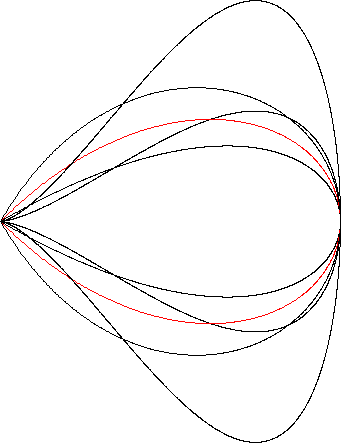Right Strophoid
RIGHT STROPHOID

Balmoral Software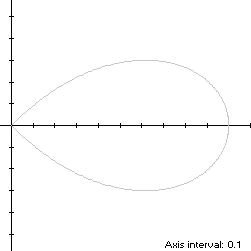The lobe of the right strophoid is a convex x-symmetric droplet-shaped closed curve S with polar equation
r(t) = 2cos(t) - sec(t), -π/4 ≤ t < π/4
The curve follows a counterclockwise path as t increases, starting from the cusp at the origin when t = -π/4, proceeding through Quadrant IV to the right edge (1,0) at t = 0, then following a symmetric path through Quadrant I back to the cusp at t = π/4. The maximum ordinate point of S is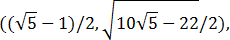so the width x height of its bounding rectangle is### Metrics

The area of S is
A = (4 - π)/2
We have
r'(t) = -2sin(t) - tan(t)sec(t),
so by (L2), the perimeter of S is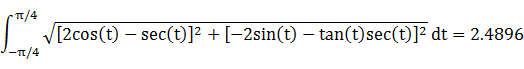By (C2), its centroid abscissa is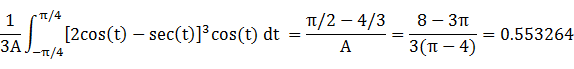### Incircle

The maximum radius of a circle centered on the x-axis and inscribed in S is its maximum ordinate, so a candidate for the incircle has radius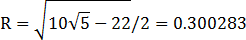and center abscissaThe candidate circle must be contained within S, so we require that c - R and c + R both be within the abscissa range [0,1] of S, which is true. For verification, we have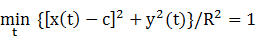### Inellipse

Using z = 1 in Lemma E,
d/dt [x(t) - z]y(t) = d/dt -2sin2(t)cos(2t)tan(t)
has a zero at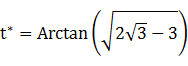The corresponding coordinates are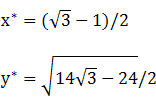We then have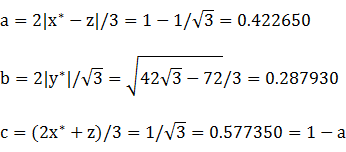For verification,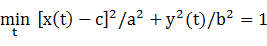### Circumellipse

Using z = 0 in Lemma E,
d/dt [x(t) - z]y(t) = d/dt cos2(2t)tan(t)
has a zero at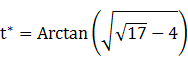The corresponding coordinates are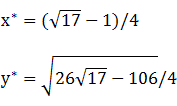We then have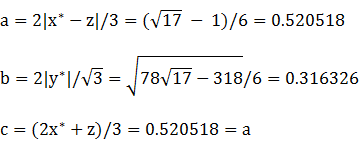For verification,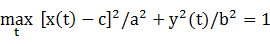### Circumcircle

Since the abscissa extrema are on the x-axis and the bounding rectangle is wider than it is tall, a candidate for the circumcircle has its radius and center abscissa both equal to 1/2. For verification, we have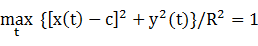### Summary Table

 Perimeter Area Centroid Figure Parameters Incircle R =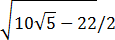1.886734 0.283277 (0.618034,0) Inellipse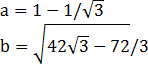2.252459 0.382312 (0.577350,0) Right strophoid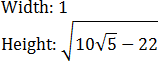2.4896 0.429204 (0.553264,0) Circumellipse2.668302 0.517274 (0.520518,0) Circumcircle R = 1/2 3.141593 0.785398 (0.5,0)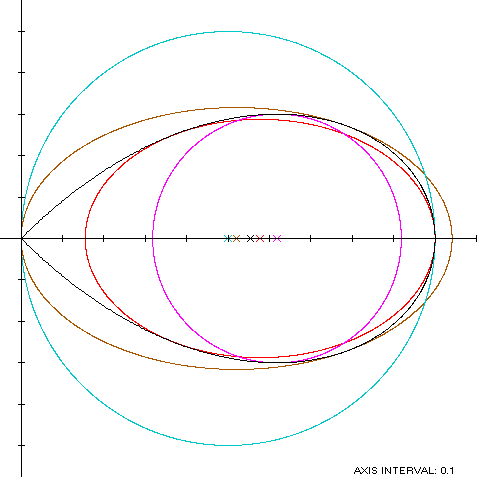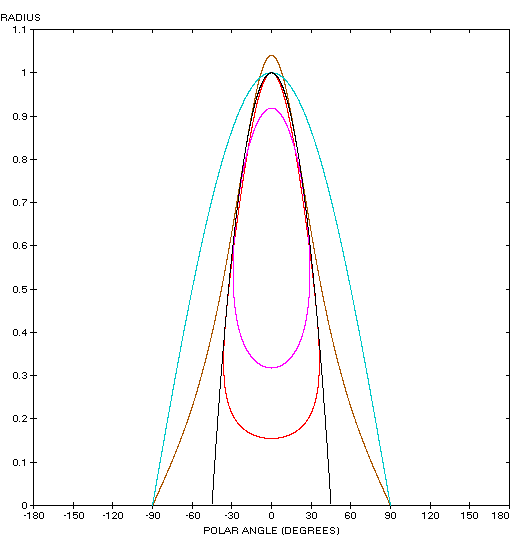The right strophoid (red) is a member of a group of similarly-shaped figures described on these pages, including (inside to outside) the teardrop curve, the Tschirnhausen cubic, the Trisectrix of Maclaurin and the piriform curve: